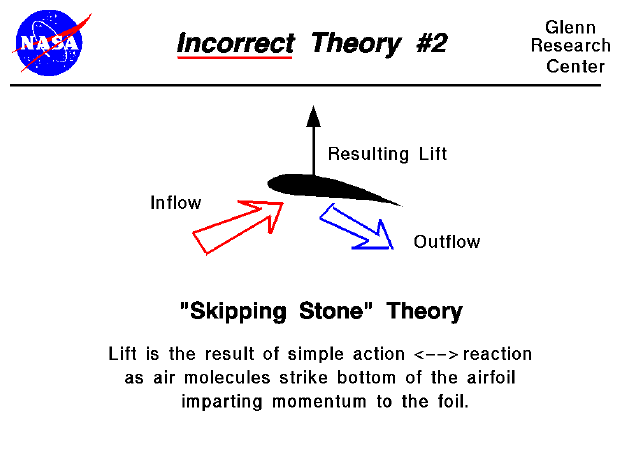There are many theories of how lift is generated. Unfortunately, many of the theories found in encyclopedias, on web sites, and even in some textbooks are incorrect, causing unnecessary confusion for students.

The theory described on this slide is often seen on web sites and in popular literature. The theory is based on the idea that lift is the reaction force to air molecules striking the bottom of the airfoil as it moves through the air. Because this is similar to the way in which a flat rock thrown at a shallow angle skips across a body of water, it is called the "Skipping Stone" theory of lift. (It is sometimes called a Newtonian theory of lift, since it involves Newton's third law, but to avoid confusion with the correct Newtonian theory of flow turning, we shall call it the "Skipping Stone" theory.)

Before considering what is wrong with this theory, let's investigate the actual flow around an airfoil by doing an experiment using a Java simulator which is solving the correct flow equations.

This interactive Java applet shows flow going past a symmetric airfoil. The flow is shown by a series of moving particles. You can change the angle of attack of the airfoil by using a slider, and the angle of attack generates the lift through flow turning. There is also a translating probe with a gage on the simulator which lets you investigate the flow.

This is a secondary Java applet which uses a text box to describe some experiments for the student to perform using the previous applet.

Let's use the information we've just learned to evaluate the "Skipping Stone" Theory.

• This theory is concerned with only the interaction of the lower surface of the moving object and the air. It assumes that all of the flow turning (and therefore all the lift) is produced by the lower surface. But as we have seen in our experiment, the upper surface also turns the flow. In fact, when one considers the downwash produced by a lifting airfoil, the upper surface contributes more flow turning the lower surface. This theory does not predict or explain this effect.
• Because this theory neglects the action <--> reaction of molecules striking the upper surface, it does not predict the negative lift present in our experiment when the angle of attack is negative. On the top of the airfoil, no vacuum exists. Molecules are still in constant random motion on the upper surface (as well as the lower surface), and these molecules strike the surface and impart momentum to the airfoil as well.
• The upper airfoil surface doesn't enter into the theory at all. So using this theory, we would expect two airfoils with the same lower surface but very different upper surfaces to give the same lift. We know this doesn't occur in reality. In fact, there are devices on many airliners called spoilers which are small plates on the upper surface, between the leading and trailing edges. They are used to change the lift of the wing to maneuver the aircraft by disrupting the flow over the upper surface. This theory does not predict or explain this effect.
• If we make lift predictions based on this theory, using a knowledge of air density and the number of molecules in a given volume of air, the predictions are totally inaccurate when compared to actual measurements. The chief problem with the theory is that it neglects the physical properties of the fluid. Lift is created by turning a moving fluid, and all parts of the solid object can deflect the fluid.

BUT..... this theory is not totally inaccurate. In certain flight regimes, where the velocity is very high and the density is very low, few molecules can strike the upper airfoil surface and the Newtonian theory gives the correct predictions. These are the conditions which occur on the Space Shuttle during the early phases of its re-entry into the Earth's atmosphere at altitudes above about 50 miles and at velocities above 10,000 mph (hypersonic conditions). For these flight conditions, the theory gives a correct prediction. However, for most normal flight conditions, like those on an airliner (35,000 feet, 500 mph), this theory does not give the right answer.Guided Tours